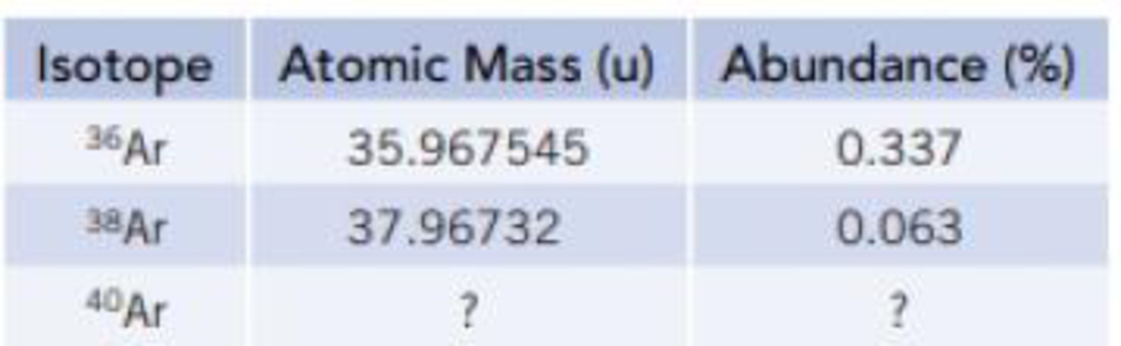# Atmospheric argon is a mixture of three stable isotopes. 36 Ar. 38 Ar, and 40 Ar. Use the information in the table below to determine the atomic mass and natural abundance of 40 Ar.### Chemistry & Chemical Reactivity

9th Edition
John C. Kotz + 3 others
Publisher: Cengage Learning
ISBN: 9781133949640

#### Solutions

Chapter
Section### Chemistry & Chemical Reactivity

9th Edition
John C. Kotz + 3 others
Publisher: Cengage Learning
ISBN: 9781133949640
Chapter 2.10, Problem 5Q
Textbook Problem
43 views

## Atmospheric argon is a mixture of three stable isotopes. 36Ar. 38Ar, and 40Ar. Use the information in the table below to determine the atomic mass and natural abundance of 40Ar.Interpretation Introduction

Interpretation: The atomic mass and natural abundance for given 40Ar has to be determined from the given data’s of other isotopes.

Concept Introduction:

The average mass of argon is 39.948 u.

Equation for average weight is,

average mass=(%abundanceisotop-1100)×(massofisotop-1) +(%abundanceisotop-2100)×(massofisotop-2)+etc

Two species are said to be isotopes if they have same atomic number but different mass number.

### Explanation of Solution

There are three isotopes for argon atom.

The mass of isotope-1 is 35.967545u and its abundance is 0.337%.

The mass of isotope-2 is 37.96732u and its abundance is 0.063%.

Therefore, abundance of isotope-3 is 100%-(0.337%+0.063%)=99.6%

Equation for average weight is,

average weight=(%abundanceisotop-1100)×(massofisotop-1) +(%abundanceisotop-2100)×(massofisotop-2)+etc

Therefore,

average weightofargon=(0.337100)×(35

### Still sussing out bartleby?

Check out a sample textbook solution.

See a sample solution

#### The Solution to Your Study Problems

Bartleby provides explanations to thousands of textbook problems written by our experts, many with advanced degrees!

Get Started

Find more solutions based on key concepts
Blood carries nutrients absorbed from food a. from the intestine to the liver. b. from the lungs to the extremi...

Nutrition: Concepts and Controversies - Standalone book (MindTap Course List)

What kind of bond between atoms is most prevalent among organic compounds?

Chemistry for Today: General, Organic, and Biochemistry

In Figure P30.26, a semicircular conductor of radius R = 0.250 m is rotated about the axis AC at a constant rat...

Physics for Scientists and Engineers, Technology Update (No access codes included)